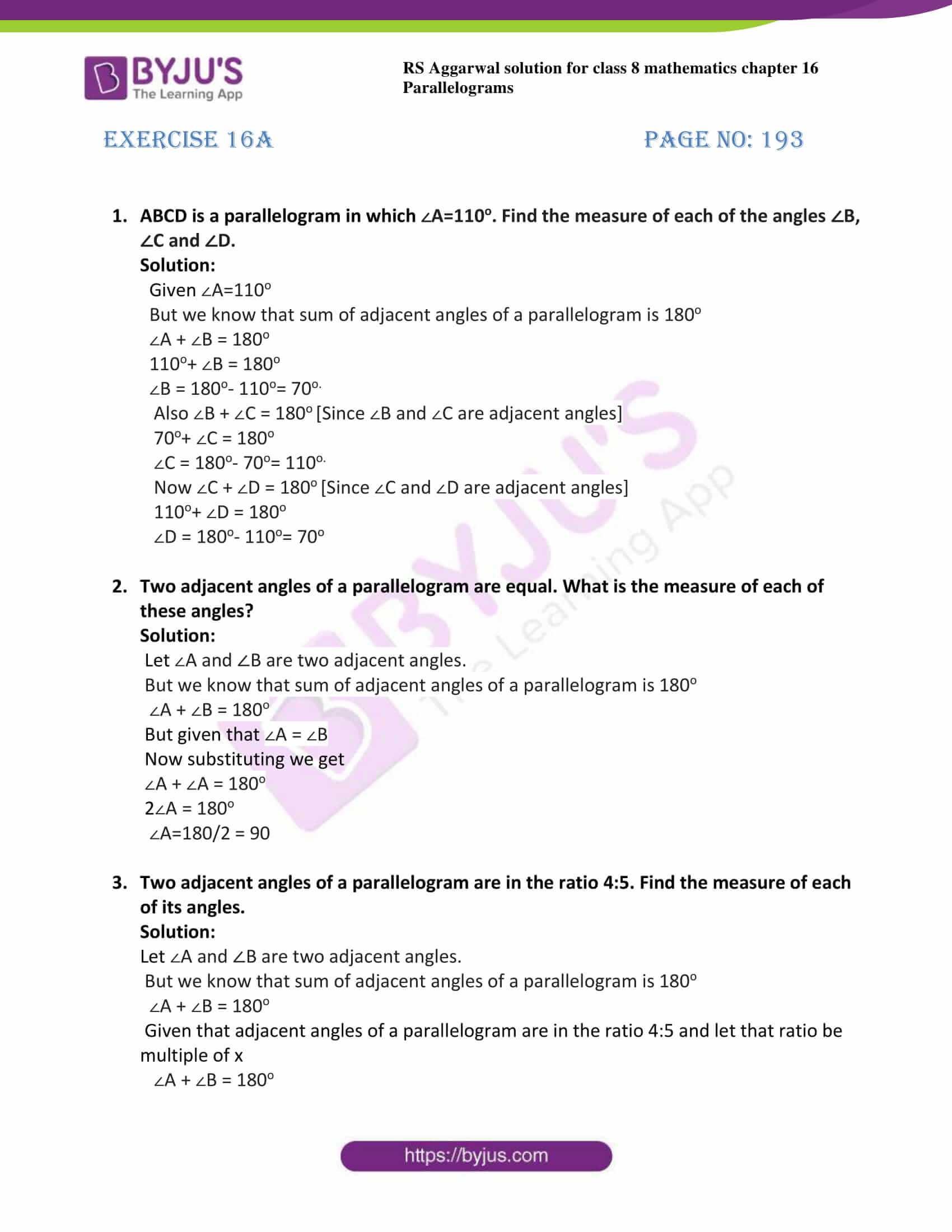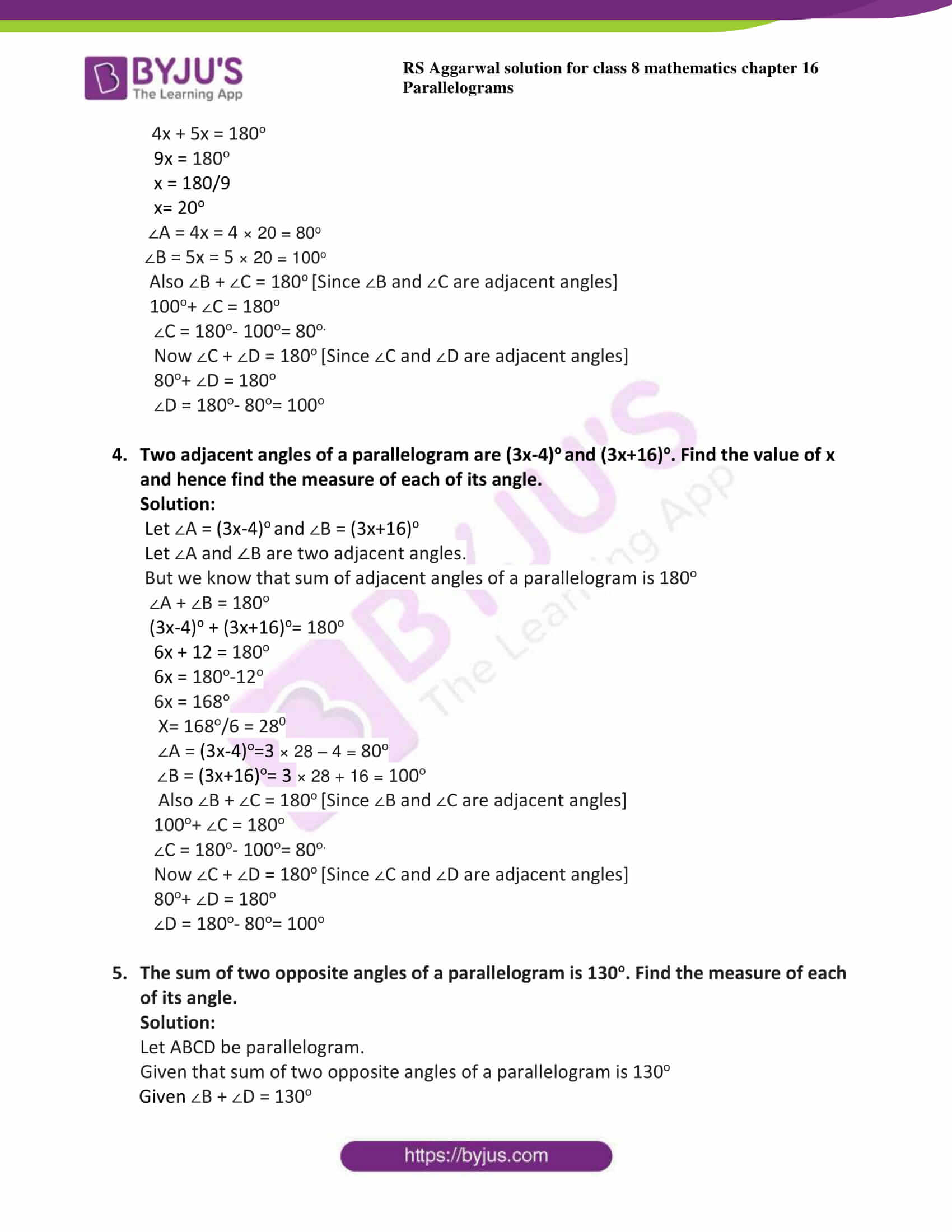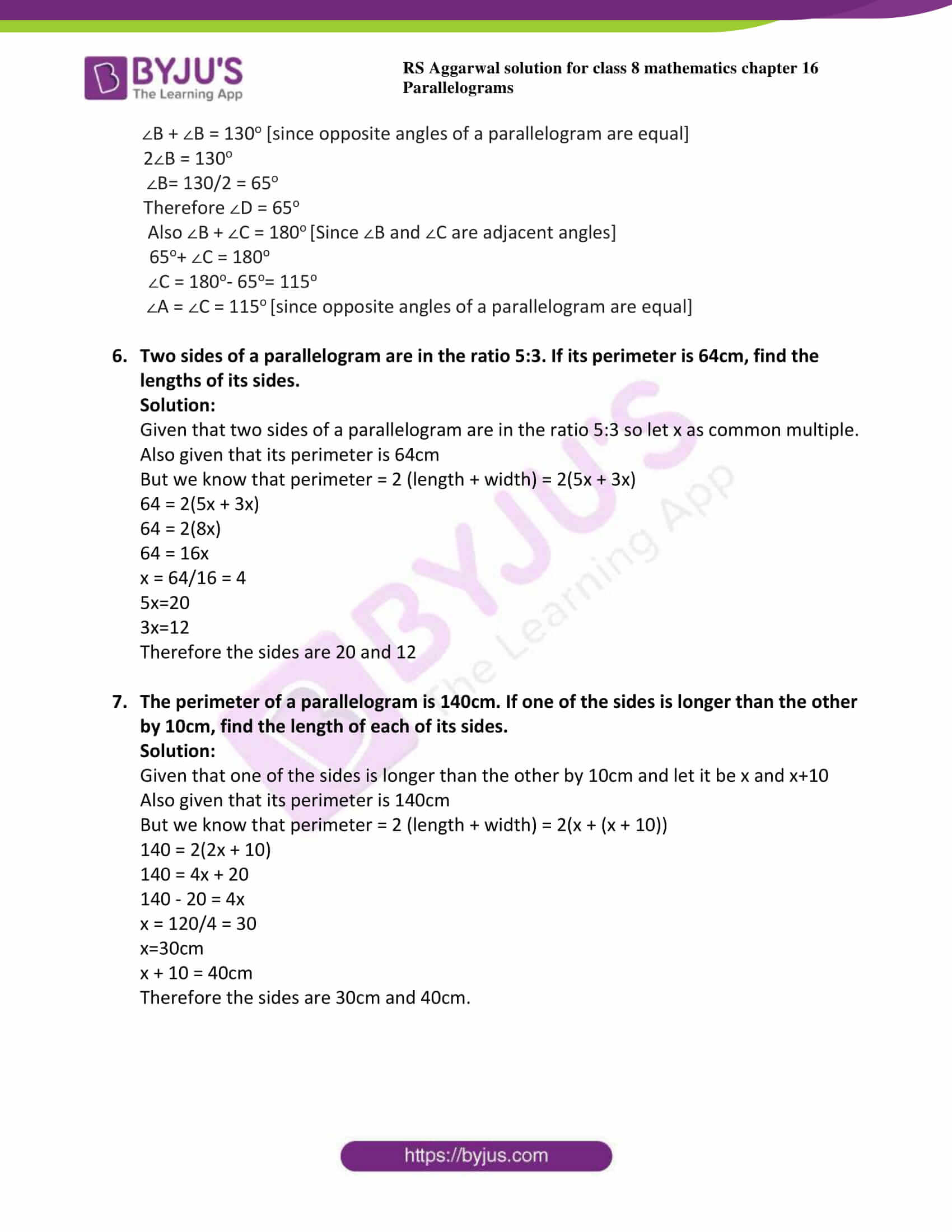# RS Aggarwal Solutions for Class 8 Maths Chapter 16 - Parallelograms Exercise 16A

Students can get the pdf of RS Aggarwal Solutions for the Exercise 16A of Class 8 Maths Chapter 16, Parallelogram from the link available here. BYJU’S expert team have solved in such a way that learners can understand easily and comfortably. This exercise includes fourteen questions based on parallelogram as well as properties of a parallelogram. By practising the RS Aggarwal Solutions for class 8, students will be able to grasp the concepts perfectly. Hence, students whose aim is to score high in the examinations are advised to go through RS Aggarwal Solutions for Class 8.

## Download PDF of RS Aggarwal Solutions for Class 8 Maths Chapter 16 – Parallelograms Exercise 16A### Access answers to Maths RS Aggarwal Solutions for Class 8 Chapter 16 – Parallelograms Exercise 16A

1. ABCD is a parallelogram in which ∠A=110o. Find the measure of each of the angles ∠B, ∠C and ∠D.

Solution:

Given ∠A=110o

But we know that sum of adjacent angles of a parallelogram is 180o

∠A + ∠B = 180o

110o+ ∠B = 180o

∠B = 180o– 110o= 70o.

Also ∠B + ∠C = 180o [Since ∠B and ∠C are adjacent angles]

70o+ ∠C = 180o

∠C = 180o– 70o= 110o.

Now ∠C + ∠D = 180o [Since ∠C and ∠D are adjacent angles]

110o+ ∠D = 180o

∠D = 180o– 110o= 70o

2. Two adjacent angles of a parallelogram are equal. What is the measure of each of these angles?

Solution:

Let ∠A and ∠B are two adjacent angles.

But we know that sum of adjacent angles of a parallelogram is 180o

∠A + ∠B = 180o

But given that ∠A = ∠B

Now substituting we get

∠A + ∠A = 180o

2∠A = 180o

∠A=180/2 = 90

3. Two adjacent angles of a parallelogram are in the ratio 4:5. Find the measure of each of its angles.

Solution:

Let ∠A and ∠B are two adjacent angles.

But we know that sum of adjacent angles of a parallelogram is 180o

∠A + ∠B = 180o

Given that adjacent angles of a parallelogram are in the ratio 4:5 and let that ratio be multiple of x

∠A + ∠B = 180o

4x + 5x = 180o

9x = 180o

x = 180/9

x= 20o

∠A = 4x = 4 × 20 = 80o

∠B = 5x = 5 × 20 = 100o

Also ∠B + ∠C = 180o [Since ∠B and ∠C are adjacent angles]

100o+ ∠C = 180o

∠C = 180o– 100o= 80o.

Now ∠C + ∠D = 180o [Since ∠C and ∠D are adjacent angles]

80o+ ∠D = 180o

∠D = 180o– 80o= 100o

4. Two adjacent angles of a parallelogram are (3x-4)o and (3x+16)o. Find the value of x and hence find the measure of each of its angle.

Solution:

Let ∠A = (3x-4)o and ∠B = (3x+16)o

Let ∠A and ∠B are two adjacent angles.

But we know that sum of adjacent angles of a parallelogram is 180o

∠A + ∠B = 180o

(3x-4)o + (3x+16)o= 180o

6x + 12 = 180o

6x = 180o-12o

6x = 168o

X= 168o/6 = 280

∠A = (3x-4)o=3 × 28 – 4 = 80o

∠B = (3x+16)o= 3 × 28 + 16 = 100o

Also ∠B + ∠C = 180o [Since ∠B and ∠C are adjacent angles]

100o+ ∠C = 180o

∠C = 180o– 100o= 80o.

Now ∠C + ∠D = 180o [Since ∠C and ∠D are adjacent angles]

80o+ ∠D = 180o

∠D = 180o– 80o= 100o

5. The sum of two opposite angles of a parallelogram is 130o. Find the measure of each of its angle.

Solution:

Let ABCD be parallelogram.

Given that sum of two opposite angles of a parallelogram is 130o

Given ∠B + ∠D = 130o

∠B + ∠B = 130o [since opposite angles of a parallelogram are equal]

2∠B = 130o

∠B= 130/2 = 65o

Therefore ∠D = 65o

Also ∠B + ∠C = 180o [Since ∠B and ∠C are adjacent angles]

65o+ ∠C = 180o

∠C = 180o– 65o= 115o

∠A = ∠C = 115o [since opposite angles of a parallelogram are equal]

6. Two sides of a parallelogram are in the ratio 5:3. If its perimeter is 64cm, find the lengths of its sides.

Solution:

Given that two sides of a parallelogram are in the ratio 5:3 so let x as common multiple.

Also given that its perimeter is 64cm

But we know that perimeter = 2 (length + width) = 2(5x + 3x)

64 = 2(5x + 3x)

64 = 2(8x)

64 = 16x

x = 64/16 = 4

5x=20

3x=12

Therefore the sides are 20 and 12

7. The perimeter of a parallelogram is 140cm. If one of the sides is longer than the other by 10cm, find the length of each of its sides.

Solution:

Given that one of the sides is longer than the other by 10cm and let it be x and x+10

Also given that its perimeter is 140cm

But we know that perimeter = 2 (length + width) = 2(x + (x + 10))

140 = 2(2x + 10)

140 = 4x + 20

140 – 20 = 4x

x = 120/4 = 30

x=30cm

x + 10 = 40cm

Therefore the sides are 30cm and 40cm.

## RS Aggarwal Solutions for Class 8 Maths Chapter 16 – Parallelograms Exercise 16A

Exercise 16A of RS Aggarwal Solutions for Chapter 16, Parallelograms deals with the topics related to special types of quadrilaterals and properties of quadrilaterals. Some of the topics focused prior to exercise 16A include the following.

• Definition of parallelogram
• Definition of rhombus
• Definition of rectangle
• Definition of square
• Definition of trapezium
• Definition of an isosceles trapezium

The RS Aggarwal Solutions can help the students in practising and learning each and every concept as it provides solutions to all questions asked in the RS Aggarwal textbook.